# Polynomials as Derivatives

Ever wondered if you could represent any polynomial in terms of only its derivatives? No? Well I did. Here's the result of that.

It all started with the desire to find roots of higher-order polynomials. As such, it started with setting the equation equal to 0 and then looking at the roots. For a first order polynomial f(x) = ax + b, that would be x = -b/a. There is a b and an a term in there. Is there a way to express this term in only forms of f(x)? Well, if you plugged in 0 for x, then you get f(0) = b, so that's the b term by itself. If you take the derivative of f(x) you get f'(x) = a, and that's the a term by itself! So, you could say that the root of a linear polynomial is x = -f(0)/(f(x). Make sense?

For now, I'm going to not set the equation equal to 0 and just convert the polynomial in its current form the best I can.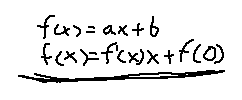This makes sense. The a value is the slope, which becomes f'(x), and the y-intercept value, b, becomes f(0), for when x = 0. Let's try a harder one. How about a more concrete example first?

``` f(x) = x^2 + 3x -1 ```

The first step is the find the derivatives of this function, and also at all the points where the equations go to 0. Calculating time!

```            ```
f(0) = -1
f'(x) = 2x + 3
f'(0) = 3
f''(x) = 2
```
```

We need to get rid of the x^2 term first, as no substitutions exist for that. Factor out the x like so:

``` f(x) = x(x + 3) - 1 ```

It almost looks like 2x + 3.. just need to multiply a 2 on both sides of the equation.

``` 2f(x) = x(2x + 6) - 2 ```

Split out the 6 into two 3's, and we can finally substitute f'(x) in there.

``` 2f(x) = x(f'(x) + 3) - 2 ```

We have substitution possibilities for the 3 and the 2: f'(0) and f''(x), respectively.

``` 2f(x) = x(f'(x) + f'(0)) - f''(x) ```

We got rid of all the constants, except for the 2, but we put that 2 in ourselves, so that's fine. Doing this for the general form nets the same result.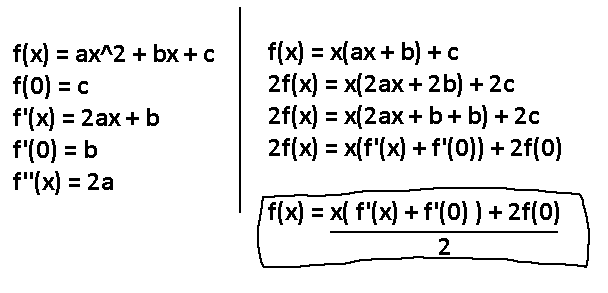We can use the same process for higher-order polynomials. Observe: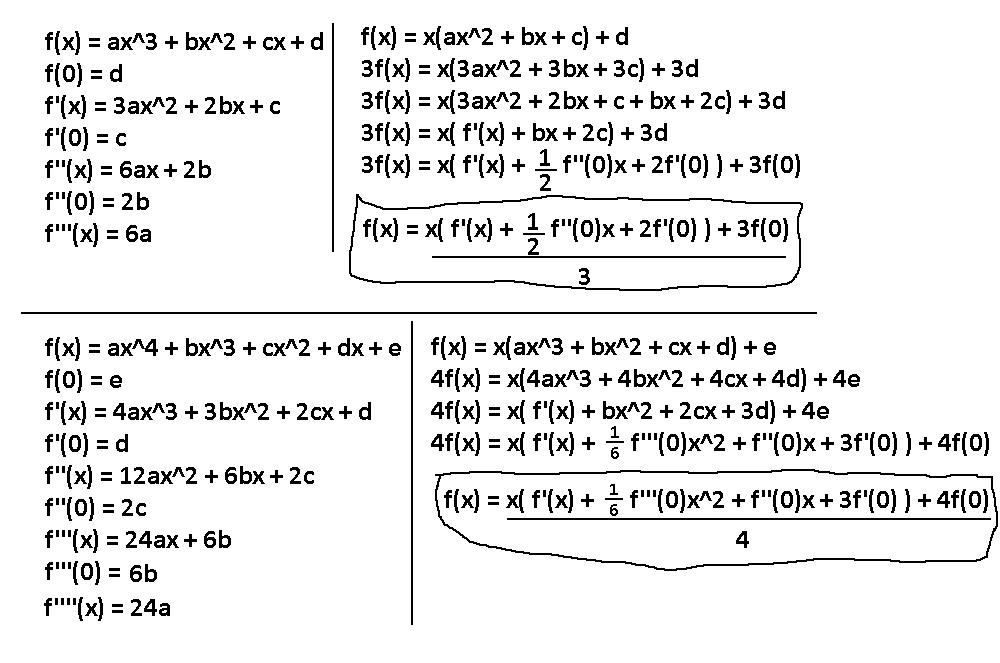Here's a summary of all the formulas so far, including an extra one I did without the work behind it: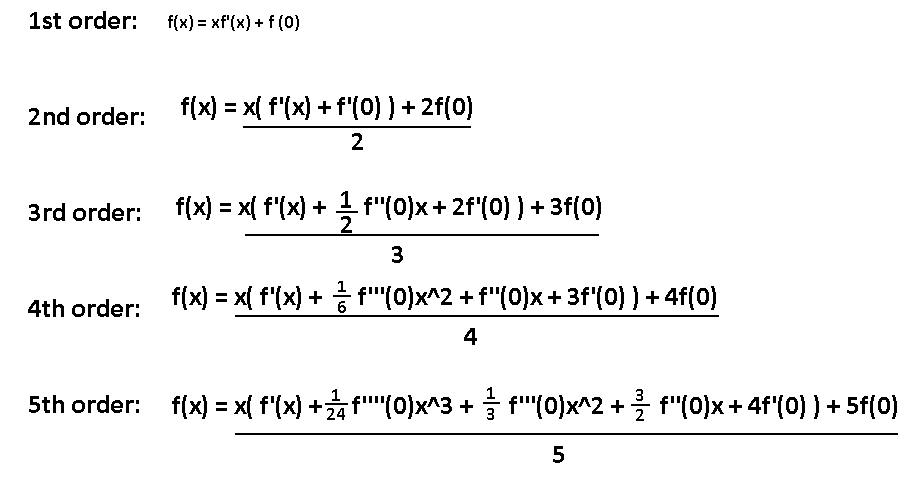I'm gonna stop here before it gets too ugly. Besides, what usually happens at this point is I go to some ridiculous length to make this whole process generic. Oh look, it's about to happen.

A pretty specific procedure happens here. Find all derivatives and when those functions have x = 0. Then, factor out an x in the original f(x) and multiply by some constant to match f'(x)'s first term. Then, substitute the terms in the parentheses with f'(x) and look at the remaining terms that fall out. Then for each remaining term, substitute in the appropriate f(0) variant and coefficient, including the final term outside the parentheses.

What so far is staying the same? Well, this general structure, for starters. Also, the denominator increases linearly with the order number, which I'll call N, as well as the coefficient of the final term.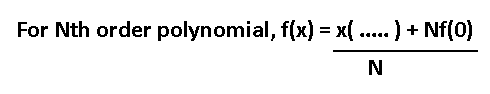So, all that's needed left is the figure out the insides of the parentheses. Easier said than done. There will be an f'(x) plus N extra terms. Recall a step in the middle of transforming these functions before all the substitutions start happening, shown below.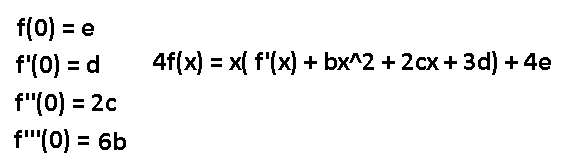This form is much easier to find the pattern. f'(x) stays there, and then all future terms have the coefficients increase linearly, while the order of the term decreases linearly. Check out the f(0) variants on the left, which would get substituted in to the equation on the right on the next step. Those terms also follow a pattern, as the coefficients increase factorially for every term. Combining all of this knowledge we are almost ready for the final step, but there is one last thing to take care of. Instead of calling our coefficient variables a, b, c, etc… I'm going to call them C1, C2, C3, etc… This way if I want to reference the Nth constant variable I can just do C(N). Also, for the nth derivative of f(x) I'm going to use fn(x) instead, so f'''(x) becomes f3(x). Okay? Okay.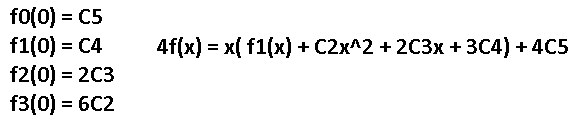This is getting tedious. Anyway, start generalizing these sequences for the Nth order polynomial so that magic can start happening.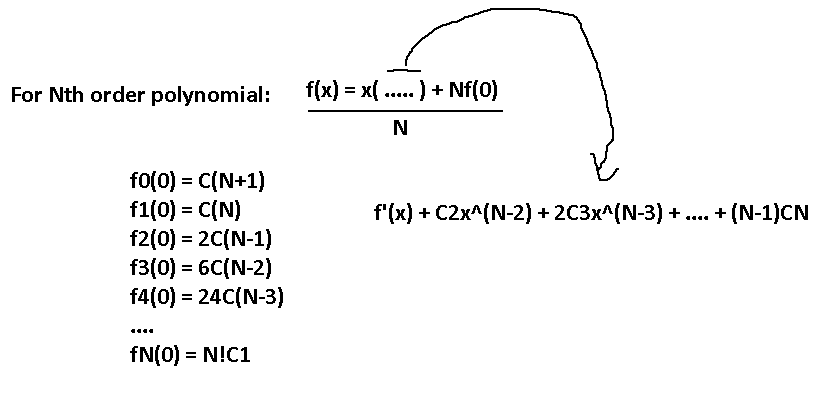Has your brain turned into putty yet? There's still the subtitution step remaining…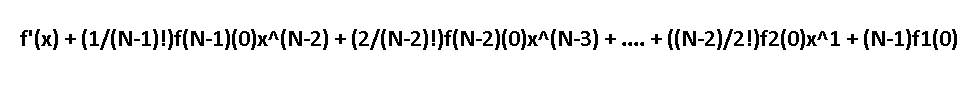Ooookay, time to clean this up. We're going to use our old pal Sigma notation to turn this into a summation to simplify things greatly.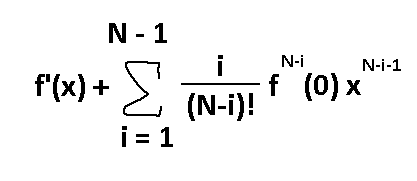Wow.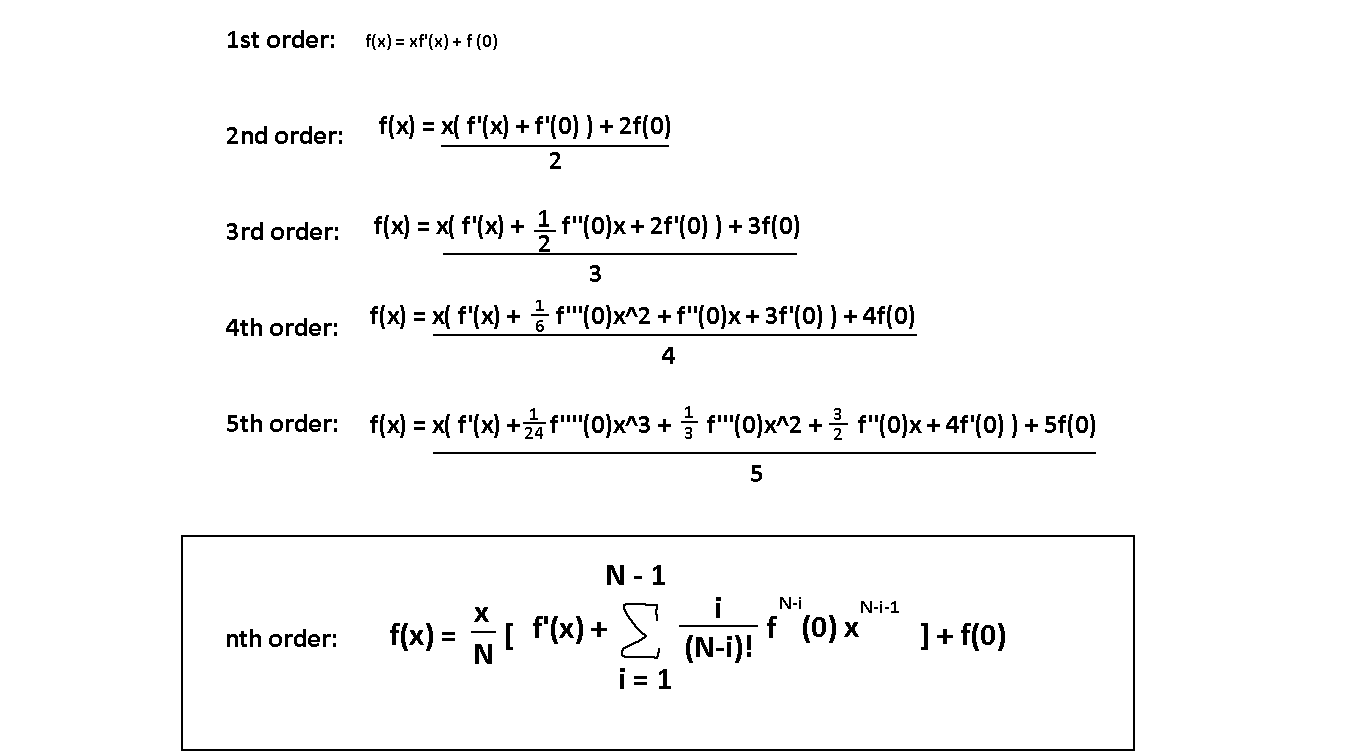What a behemoth. Plug in N = 1, and you'll get back the first order formula. Since the summation will go from i = 1 to i = 0, that whole summation part will give you 0 since the range isn't valid. Plug in N = 2, and you'll get your 2nd order formula. And so on…

Yeah, I think it's time to stop.